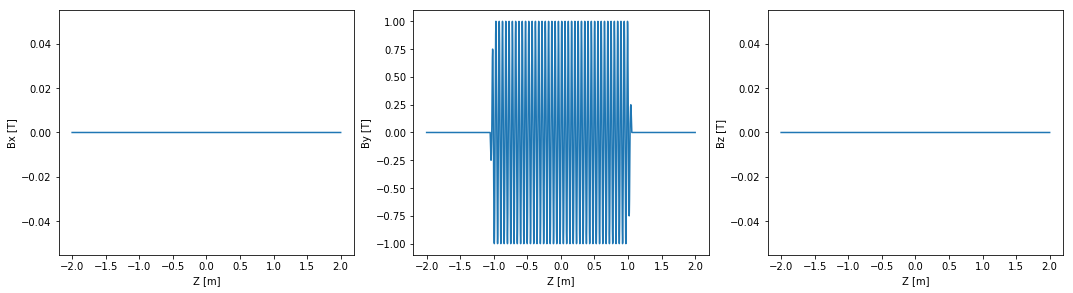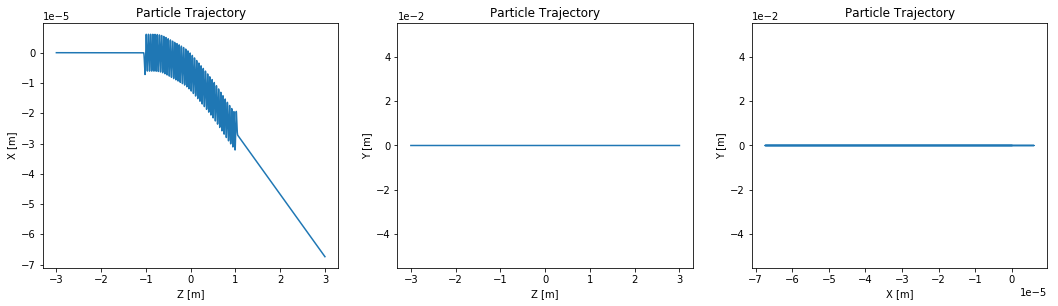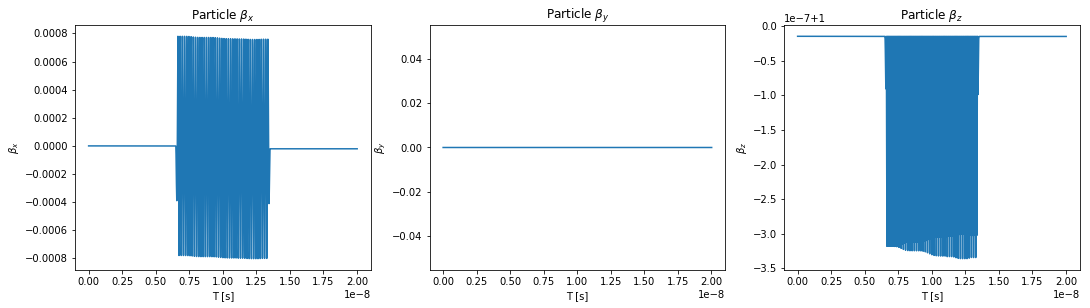# Example 006: Imported Field Trajectory¶

In this example the trajectory is calculated for a field coming from an imported data file. We first creat the data file by adding some undulator field, some noise, and exporting the field to a file. Several formats are possible (for more information see the All About Magnetic Fields tutorial.

In :
# This has nothing to do with OSCARS, but it puts the matplotlib plots inline in the notebook
%matplotlib inline

# Import the OSCARS SR module
import oscars.sr

# Import basic plot utilities (matplotlib).  You don't need these to run OSCARS, but it's used here for basic plots
from oscars.plots_mpl import *

OSCARS v2.1.8 - Open Source Code for Advanced Radiation Simulation
Brookhaven National Laboratory, Upton NY, USA
http://oscars.bnl.gov
oscars@bnl.gov

In :
# Create a new OSCARS object.  Default to 8 threads and always use the GPU if available


## Create a Magnetic field and Export it to a file¶

We are only doing this so you don't have to download many data files.

In :
# Clear any existing fields (just good habit in notebook style) and add an undulator field
osr.clear_bfields()
osr.add_bfield_undulator(bfield=[0, 1, 0], period=[0, 0, 0.049], nperiods=41)

for i in range(10):
bfield=[0, 0.001 * (osr.rand() - 0.5), 0],
sigma=[0, 0, 0.1*(osr.rand())],
translation=[0, 0, 2 * (osr.rand() - 0.5)]
)

# Export the field
osr.write_bfield(ofile='EX006.dat', oformat='OSCARS', zlim=[-3, 3], nz=50000)


## Import the magnetic field¶

In :
# Clear all magnetic fields!
osr.clear_bfields()

# Import the field from the file created above

# Plot imported field
plot_bfield(osr, -2, 2)Here we add a particle beam making use of some of the defaults, namely:

* type='electron'
* t0=0
* d0=[0, 0, 1]



One must specify ctstartstop. This is the start and stop time of the calculation. In this example we will start the calculation at t=0 and go to t=6 (given in units of ct) since the beam is relativistic. In this example you can specify the start time as less than 0 which is useful if you want to propogate the particle backwars in time. This is useful for instance if you have a bending magnet before the undulator that you wish to include.

clear_particle_beams() is called, again for convenience, but it is not necessary.

In :
# Setup beam similar to NSLSII with different starting position from above
# (this makes more sense for some scenarios)
osr.clear_particle_beams()
osr.set_particle_beam(x0=[0, 0, -3], energy_GeV=3, current=0.500)

# Set the start and stop times for the calculation
osr.set_ctstartstop(0, 6)


## Calculate Trajectory¶

Now we calculate the trajectory and plot it. It is enough to call calculate_trajectory(). If you are doing other calculations (flux, spectra, power density) it is not necesary to call this since it is called internally.

In :
# Run the particle trajectory calculation
trajectory = osr.calculate_trajectory()

# Plot the trajectory position and velocity
plot_trajectory_position(trajectory)
plot_trajectory_velocity(trajectory)